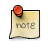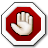Navigation C API Pages Python bindings Applications

## Vector

Vector is a resizeable C array.

Vector expects a frequent resizes hence it keeps the underlying memory size slightly bigger than actual size in order to keep the memory reallocations at minimum.See also vector example usage.
Vector allocation
```void *gp_vec_new(size_t length, size_t unit);

/* Example usage */
struct user_struct {
...
};

void func(...)
{
struct user_struct *array = gp_vec_new(10, sizeof(struct user_struct));

if (!array)
return;

array.foo = 123;

...

gp_vec_free(array);
}```

Allocates a new vector of initial `length` and for elements of size `unit`.

Initial length can be 0 as vector can be resized later.

Returns NULL on allocation failure.

Vector copy
`void *gp_vec_dup(void *self);`

Allocates a new vector of the same size and unit as `self` and copies the data.

Returns copy of a vector on NULL on allocation failure.

Vector free
`void gp_vec_free(void *self);`

Frees the vector. Passing NULL as `self` is no-op.

Vector lenght and unit
```size_t gp_vec_len(const void *self);

size_t gp_vec_unit(const void *self);```

The `gp_vec_len()` returns vector length i.e. number of elements in the vector.

The `gp_vec_size()` returns vector element size i.e. number of bytes each vector element occupies.

Vector resize
```void *gp_vec_resize(void *self, size_t length);

void *gp_vec_expand(void *self, size_t lenght);

void *gp_vec_shrink(void *self, size_t lenght);

/* Example usage */
int *func(int *array, int append)
{
size_t last = gp_vec_len(array);

int *new = gp_vec_append(self, 1);
if (!new)
return NULL;

new[last] = append;

return new;
}```

Resizes a vector.

If space was added after the vector it’s filled with zeroes.

The call may return NULL if we attempted to add space to the vector and allocation has failed, in this case the original vector is kept untouched.

Vector shrinking cannot fail.Returns a new pointer to the vector!
Vector insert
```void *gp_vec_ins(void *self, size_t off, size_t length);

/* Example usage */
int *func(int *array, int prepend)
{
int *new = gp_vec_ins(self, 0, 1);
if (!new)
return NULL;

new = prepend;

return new;
}```

Inserts a gap into the vector, the newly inserted gap is filled with zeroes.

The call may return NULL if underlying memory allocation has failed, in this case the original vector is kept untouched.

Returns NULL if `off` is outside of the vector.Returns a new pointer to the vector!
Vector delete
```void *gp_vec_del(void *self, size_t off, size_t length);

/* Example usage */
int *func(int *array, int *pop)
{
int *new;

*pop = array;

new = gp_vec_del(self, 0, 1);
if (!new)
return NULL;

return new;
}```

Removes a range from the vector.

Returns NULL if the block is outside of the vector.Returns a new pointer to the vector!